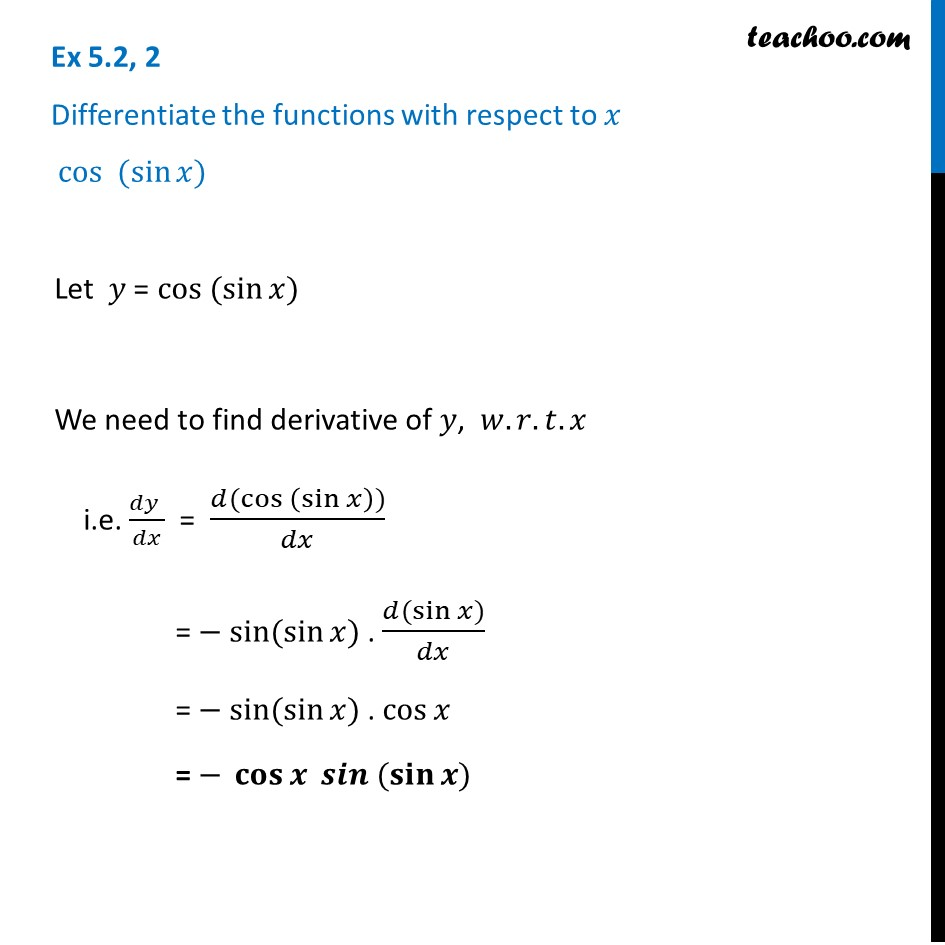Finding derivative of a function by chain rule

Chapter 5 Class 12 Continuity and Differentiability (Term 1)
Concept wise### Transcript

Ex 5.2, 2 Differentiate the functions with respect to 𝑥 cos (sin⁡𝑥) Let 𝑦 = cos (sin⁡𝑥) We need to find derivative of 𝑦, 𝑤.𝑟.𝑡.𝑥 i.e. (𝑑𝑦 )/𝑑𝑥 = (𝑑(cos (sin⁡𝑥 )))/𝑑𝑥 = − sin⁡(sin⁡𝑥) . (𝑑(sin⁡〖𝑥)〗)/𝑑𝑥 = − sin⁡(sin⁡𝑥) . cos⁡𝑥 = − 𝐜𝐨𝐬⁡𝒙 𝒔𝒊𝒏 ⁡(𝐬𝐢𝐧⁡〖𝒙)〗